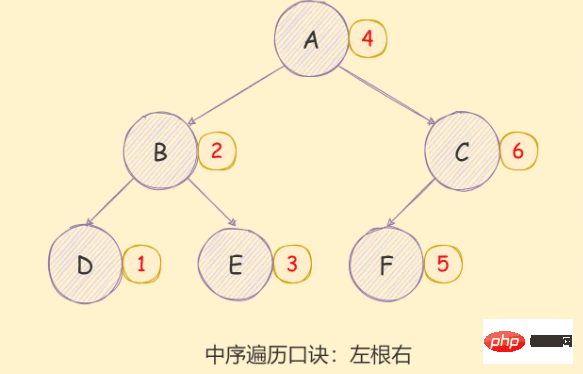# 详细介绍JavaScript二叉树及各种遍历算法【相关推荐：javascript视频教程web前端

## 什么是二叉树• `i`层的节点最有只有`2^(i-1)`个；
• 如果这颗二叉树的深度为`k`，那二叉树最多有`2^k-1`个节点；
• 在一个非空的二叉树中，若使用`n0`表示叶子节点的个数，`n2`是度为2的非叶子节点的个数，那么两者满足关系`n0 = n2 + 1`

### 满二叉树• 满二叉树的的第`n`层具有`2^(n-1)`个节点；
• 深度为`k`的满二叉树一定存在`2^k-1`个节点，叶子节点的个数为`2^(k-1)`
• 具有`n`个节点的满二叉树的深度为`log_2^(n+1)`

### 完全二叉树## 二叉树的存储

### 数组存储### 链表存储## 与二叉树相关的算法

```// tree.js
const bt = {
val: 'A',
left: {
val: 'B',
left: { val: 'D', left: null, right: null },
right: { val: 'E', left: null, right: null },
},
right: {
val: 'C',
left: {
val: 'F',
left: { val: 'H', left: null, right: null },
right: { val: 'I', left: null, right: null },
},
right: { val: 'G', left: null, right: null },
},
}
module.exports = bt```

### 深度优先遍历

• 访问根节点；
• 访问根节点的`left`
• 访问根节点的`right`
• 重复执行第二三步

```const bt = {
val: 'A',
left: {
val: 'B',
left: { val: 'D', left: null, right: null },
right: { val: 'E', left: null, right: null },
},
right: {
val: 'C',
left: {
val: 'F',
left: { val: 'H', left: null, right: null },
right: { val: 'I', left: null, right: null },
},
right: { val: 'G', left: null, right: null },
},
}

function dfs(root) {
if (!root) return
console.log(root.val)
root.left && dfs(root.left)
root.right && dfs(root.right)
}
dfs(bt)
/** 结果
A B D E C F H I G
*/```

### 广度优先遍历

• 创建队列，把根节点入队
• 把对头出队并访问
• 把队头的`left``right`依次入队
• 重复执行2、3步，直到队列为空

```function bfs(root) {
if (!root) return
const queue = [root]
while (queue.length) {
const node = queue.shift()
console.log(node.val)
node.left && queue.push(node.left)
node.right && queue.push(node.right)
}
}
bfs(bt)
/** 结果
A B C D E F G H I
*/```

### 先序遍历

• 访问根节点;
• 对当前节点的左子树进行先序遍历；
• 对当前节点的右子树进行先序遍历；```const bt = require('./tree')

function preorder(root) {
if (!root) return
console.log(root.val)
preorder(root.left)
preorder(root.right)
}
preorder(bt)
/** 结果
A B D E C F H I G
*/```

```// 非递归版
function preorder(root) {
if (!root) return
// 定义一个栈，用于存储数据
const stack = [root]
while (stack.length) {
const node = stack.pop()
console.log(node.val)
/* 由于栈存在先入后出的特性，所以需要先入右子树才能保证先出左子树 */
node.right && stack.push(node.right)
node.left && stack.push(node.left)
}
}
preorder(bt)
/** 结果
A B D E C F H I G
*/```

### 中序遍历

• 对当前节点的左子树进行中序遍历；
• 访问根节点;
• 对当前节点的右子树进行中序遍历；```const bt = require('./tree')

// 递归版
function inorder(root) {
if (!root) return
inorder(root.left)
console.log(root.val)
inorder(root.right)
}
inorder(bt)

/** 结果
D B E A H F I C G
*/```

```// 非递归版
function inorder(root) {
if (!root) return
const stack = []
// 定义一个指针
let p = root
// 如果栈中有数据或者p不是null，则继续遍历
while (stack.length || p) {
// 如果p存在则一致将p入栈并移动指针
while (p) {
// 将 p 入栈，并以移动指针
stack.push(p)
p = p.left
}

const node = stack.pop()
console.log(node.val)
p = node.right
}
}
inorder(bt)
/** 结果
D B E A H F I C G
*/```

### 后序遍历

• 对当前节点的左子树进行后序遍历；
• 对当前节点的右子树进行后序遍历；
• 访问根节点;```const bt = require('./tree')

// 递归版
function postorder(root) {
if (!root) return
postorder(root.left)
postorder(root.right)
console.log(root.val)
}
postorder(bt)
/** 结果
D E B H I F G C A
*/```

```// 非递归版
function postorder(root) {
if (!root) return
const outputStack = []
const stack = [root]
while (stack.length) {
const node = stack.pop()
outputStack.push(node)
// 这里先入left需要保证left后出，在stack中后出，就是在outputStack栈中先出
node.left && stack.push(node.left)
node.right && stack.push(node.right)
}
while (outputStack.length) {
const node = outputStack.pop()
console.log(node.val)
}
}
postorder(bt)
/** 结果
D E B H I F G C A
*/```

【相关推荐：javascript视频教程web前端1/1